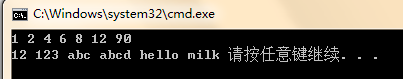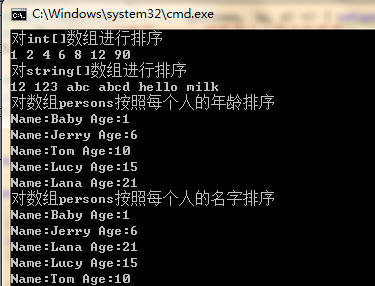遇到问题应该多思考一下——由一个泛型方法想到的

public class SortHelper
{
public void BubbleSort<T>(T[] array)
{
if (array==null)
{
throw new ArgumentNullException("array");
}

T temp = default(T);
for (int i = 0; i < array.Length; i++)
{
for (int j = i+1 ; j < array.Length; j++)
{

if (array[i]>=array[j])
{
temp = array[i];
array[i] = array[j];
array[j] = temp;
}
}
}
}
}

public class SortHelper
{
public static void BubbleSort<T>(T[] array) where T:IComparable<T>
{
if (array==null)
{
throw new ArgumentNullException("array");
}

T temp = default(T);
for (int i = 0; i < array.Length; i++)
{
for (int j = i+1 ; j < array.Length; j++)
{

if (array[i].CompareTo(array[j])>=0)
{
temp = array[i];
array[i] = array[j];
array[j] = temp;
}
}
}
}
}

class Program
{
static void Main(string[] args)
{
//int,double,float,string,byte等基元类型都是实现了IComparable接口的
int[] arrayInt=new int[]{4,2,8,12,6,90,1};
string[] arrayStr=new string[]{"abc","abcd","123","12","milk","hello"};
SortHelper.BubbleSort<int>(arrayInt);
SortHelper.BubbleSort<string>(arrayStr);
OutPut(arrayInt);
Console.WriteLine();
OutPut(arrayStr);
}

static void OutPut<T>(T[] array)
{
foreach (T element in array)
{
Console.Write(element);
Console.Write(" ");
}
}
}1、由于约束了T必须实现接口IComparable<T>,所以我们无法为没有实现该接口的对象进行排序，这一点使这个泛型排序方法的适用范围大大缩小了，而我们写泛型方法的初衷就是算法的重用，希望使其对尽可能多的类型都可以使用这个方法来进行排序。

2、由于要求类型T实现IComparable<T>接口,该接口中的方法也只能被实现一次,但是我们在对一个类型进行排序的时候,可能会按照种规则来进行排序,比如对象有A和B两个属性,有时候希望按照A属性的排序规则来排序,有时候希望按照B属性的排序规则来排序,这时候上面的泛型排序方法就显得不够灵活了.

public class SortHelper
{
//参数param1GreaterThanParam2用于判断第一个参数是不是比第二个参数大
public static void BubbleSort<T>(T[] array,Func<T,T,bool> param1GreaterThanParam2)
{
if (array==null)
{
throw new ArgumentNullException("array");
}

T temp = default(T);
for (int i = 0; i < array.Length; i++)
{
for (int j = i+1 ; j < array.Length; j++)
{
if (param1GreaterThanParam2(array[i],array[j]))
{
temp = array[i];
array[i] = array[j];
array[j] = temp;
}
}
}
}
}

class Program
{
static void Main(string[] args)
{
int[] arrayInt=new int[]{4,2,8,12,6,90,1};
string[] arrayStr=new string[]{"abc","abcd","123","12","milk","hello"};
SortHelper.BubbleSort<int>(arrayInt, (x, y) => { return x > y; });
SortHelper.BubbleSort<string>(arrayStr, (x, y) => { return x.CompareTo(y)>0; });
Console.WriteLine("对int[]数组进行排序");
OutPut(arrayInt);
Console.WriteLine();

Console.WriteLine("对string[]数组进行排序");
OutPut(arrayStr);
Console.WriteLine();

Person[] persons=new Person[]
{
new Person(){Age=10,Name = "Tom"},
new Person(){Age = 6,Name = "Jerry"},
new Person(){Age = 15,Name = "Lucy"},
new Person() {Age = 21,Name = "Lana"},
new Person(){Age=1,Name="Baby"}
};
SortHelper.BubbleSort<Person>(persons, (x, y) => { return x.Age > y.Age; });
Console.WriteLine("对数组persons按照每个人的年龄排序");
OutPut(persons,true);

SortHelper.BubbleSort<Person>(persons,(x,y)=> { return x.Name.CompareTo(y.Name)>0; });
Console.WriteLine("对数组persons按照每个人的名字排序");
OutPut(persons,true);
}

static void OutPut<T>(T[] array,bool newLineForEach=false)
{
foreach (T element in array)
{
Console.Write(element);
if (newLineForEach)
{
Console.WriteLine();
}
else
{
Console.Write(" ");
}
}
}
}posted @ 2012-11-28 22:47 Lukexywang 阅读(...) 评论(...) 编辑 收藏